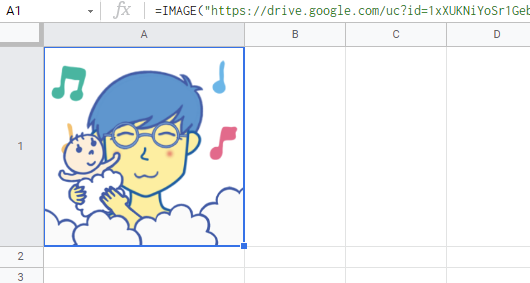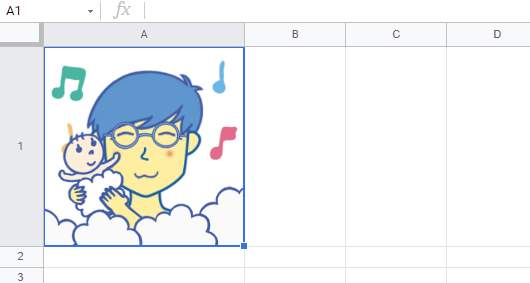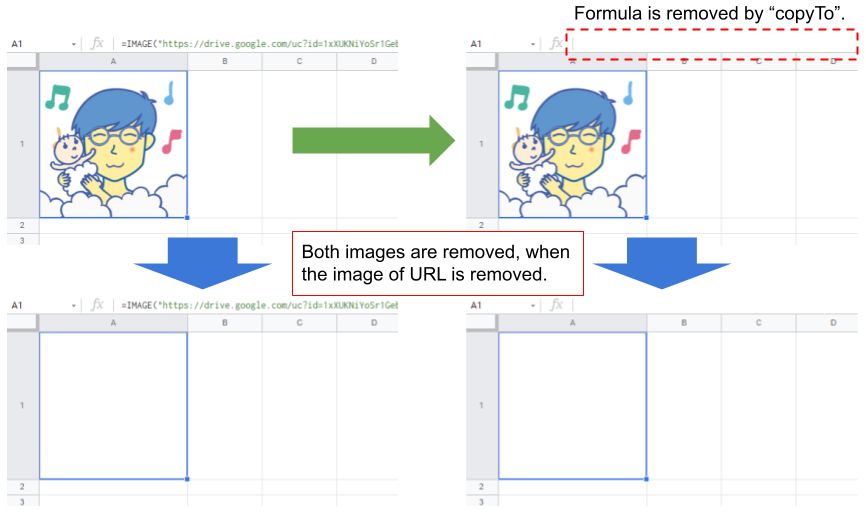Gists

### Experiment

When “=IMAGE(IMAGE_URL)” is put to a cell “A1” on Spreadsheet, the image is shown in the cell as shown in the following image.For this situation, when the following script is run,

const sheet = SpreadsheetApp.getActiveSpreadsheet().getSheetByName("Sheet1");
const range = sheet.getRange("A1");
range.copyTo(range, { contentsOnly: true });


The following result is obtained. In this case, the formula is removed and an image can be seen as shown in the following image.I thought that when the image of the URL is removed, how are those images.

### Result

As the result, it was found that when the image of the URL is removed, both images are also removed as shown in the following image.By this, it is considered that even when the formula is removed by copyTo, the image was put with the formula. On the other hand, when the image is put to the Spreadsheet using the method of insertImage, even when the image of the URL is removed, the image is not removed.

From this result, when you want to save the image from the URL, to use the method of insertImage is suitable rather than “=IMAGE(IMAGE_URL)”.